Research Projects for Potential Ph.D Students and Collaborators
Computational Mathematics Research Group (some photos)Liverpool: Prof Ke Chen, Dr Brian Doman, Dr Martyn D Hughes, Dr Yalin Zheng,
Mr Bryan Williams, Ms Lavdie Rada
Dalian: Mr Jianping Zhang, Ms Fenlin Yang and Ms Jin Zhang, Prof Bo Yu

Recent members: Dr Jon Gibson, Dr Andy Sunderland, Dr Judy M Ford, Dr Stuart Hawkins, Dr Anwar Hussein,
Dr Kamal Shaanazari, Miss Q Xie, Dr Joe Savage, Dr Noor Badshah, Dr Carlos Brito, Dr Noppadol Chumchob

Seminar links: at Maths/Liverpool :: Biocs/Liverpool :: Maths/Manchester :: POL/Liverpool :: Maths/Chester

2006 --- Fast iterative methods for solving large systems arising from variational models in image processing
by Dr Joe Savage
2008 --- Fast Iterative Methods for Variational Models in Image Segmentation
2010 --- Fast Numerical Algorithms for High Order Partial Differential Equations with Applications to Image Restoration Techniques
by Dr Carlos Francisco Brito Loeza
2010 --- A Study of Effective Variational Models and Efficient Numerical Methods for Image Registration
2012 --- Some Effective Numerical Methods for High Order Variational Models for Image Restoration
by Fenlin Yang
2012 --- PDE-based Image Segmentation and Image Denoising
by Jianping Zhang

Computational Mathematics Research Group is one of the most active research groups.
Through CMIT, all research projects within the group are directly linked to an external institution
(local hospitals, NHS centres, other sciences' departments).
We carry out research work in several aspects relating to the development of new and novel
♦ Effective Variational Models and Efficient Numerical Methods for Solving Inverse Problems

Related to the above topic, several fields are studied

and examples of a PhD project are
• Image deblurring models and applications
• Optimization methods for nonconvex models from image processing
• Variational models for combined segmentation and registration
• Modelling image fusion of 2D slices with 3D volumetric data
• Multigrid algorithms for nonlinear PDEs from imaging
• Tensor algorithms for modelling spatially variant blurs
covering various modern models and new tasks from High Resolution Image Processing (essentially we solve nonlinear partial differential equations and nonlinear optimization)
and collaborations through the Centre for Mathematical Imaging Techniques. Other recent interests includeR1. Iterative Methods for Solving Linear Systems and Related Problems R2. Numerical Solution of Boundary Integral Equations and Related Problems R3. Parallel Scientific Computing R4. Adaptive Solution of Differential Equations R5. Novel mesoscale particle modelling methods for fluids R6. Bifurcation Methods for Nonlinear Power Equations

Click to view an image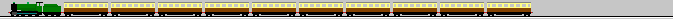...```

```

##Iterative Methods for Solving Linear Systems and Related Problems

The numerical solution of partial differential equations and integral equations in modelling various physical phenomena often requires the solution of large systems (either sparse or dense) of linear algebraic equations (either structured or unstructured). The direct solution of these linear systems using Gaussian elimination is prohibitively expensive in terms of both time and storage, so iterative methods are used. These do not require storage of the matrix but only the ability to perform matrix-vector multiplications. An important area of research is to find efficient iterative methods that require only a small number of matrix-vector multiplications and a small amount of additional work to compute a good approximate solution to the linear system.

Recent progress has been made in designing new sparse preconditioners for conjugate gradient method and the minimal residual method for linear systems. Current work deals with nonsymmetric problems. This study involves Eigenvalues, Singular value decompositions, Operator splitting, Wavelets, Various applications that include the fascinating subject of image deblurring and denoising. (See who cares about Ax=b ? and also Recent EPSRC grant)
References:

1. 1999. Discrete wavelet transforms accelerated sparse preconditioners for dense boundary element systems, by Ke Chen, Electronic Transactions on Numerical Analysis, V.8, pp. 138-153.
2. 2000. A new wavelet transform preconditioner for iterative solution of elastohydrodynamic lubrication problems. by Judy Ford, Ke Chen and Laurence Scales, International Journal of Computer Mathematics, vol.75, pp.497-513.
3. 2001. Wavelet-based preconditioners for dense matrices with non-smooth local features, by Judy Ford and Ke Chen, BIT (J. Numerical Mathematics), 41, (2), 282-307.
4. 2001. An analysis of sparse approximate inverse preconditioners for boundary integral equations, by Ke Chen, SIAM J. Matr. Anal. Appl., 22, (3), 1058-1078.
5. 2001. Wavelet-based preconditioning of dense linear systems , by J. M. Ford, Ph.D thesis, University of Liverpool, UK.
6. 2002. On two variants of an algebraic wavelet preconditioner, by Tony F. Chan and Ke Chen., SIAM Journal on Scientific Computing, 24, (1), pp.260-283.
7. 2005. A two-level sparse approximate inverse preconditioner for unsymmetric matrices, by Ke Chen and Martyn D. Hughes, IMA Journal of Numerical Analysis, Vol 26 (1), pp.11-24. [Download Paper (in pdf.gz) ]

##Numerical Solution of Boundary Integral Equations and Related Problems

Most scientific problems are formulated as partial differential equations which must be solved numerically. Sometimes the solution can be expressed as a boundary integral involving an unknown density function which can also be computed numerically by solving an integral equation. Thanks to recently developed algorithms such as iterative linear system solvers and the fast multipole method it is sometimes more efficient to solve the integral equation. Recent work has dealt with the numerical solution of integral equations and stable and efficient methods for evaluating the solution at various points once the integral representation in known. A typical problem is to compute the scattered acoustic field induced by an incident wave passing a bounded obstacle. The procedure for solving the problem is to discretize the surface of the region using triangles, solve a boundary integral equation to determine an integral expression for the solution, use this expression to evaluate the discrete Helmholtz equation at the mesh points. Fine resolution may require solution of large linear systems and often iterative solvers.
References:
1. 1991. Conjugate gradient methods for the solution of boundary integral equations on a piecewise smooth boundary, by K. Chen, Journal of Computational Physics, 97 (1), 127-143.
2. 1993. Numerical Analysis of Boundary Integral Solution of the Helmholtz equation in domains with non-smooth boundaries, by K. Chen and S. Amini, IMA J. Numer. Anal., V13, 43-66, 1993.
3. 2000. A general DRBEM model for wave refraction and diffraction, by S P Zhu, H W Liu and K Chen, J. Engineering Analysis with Boundary Elements, vol.24, No.5, pp.377-390.
4. 2001. Efficient preconditioners for iterative solution of the boundary element equations for the three dimensional Helmholtz equation, by Ke Chen and Paul Harris, Journal of Applied Numerical Mathematics, vol 36, pp.475-489.
5. 2003. On efficient preconditioners for iterative solution of a Galerkin boundary element equation for the three dimensional exterior Helmholtz problem, by Paul Harris and Ke Chen, Journal of Computational and Applied Mathematics, vol. 141. [Download Paper (in ps.gz)]
6. 2005. An implicit wavelet approximate inverse preconditioner, by Stuart C. Hawkins and Ke Chen, SIAM Journal on Scientific Computing, Vol 27, Number 2, pp. 667-686. [Download Paper (in ps.gz) ]

##Parallel Scientific Computing

The efficiency of numerical algorithms used to be measured by the number of operations (additions, subtractions, multiplications, and divisions) required. Today, this may no longer give a reasonable estimate of the relative performance of the methods. Data locality and potential for parallelism (the ability to make effective use of multiple processors simultaneously) are important properties of any numerical algorithm.

Thus linear algebra algorithms may have to re-designed and implemented in such a way as to minimize memory references and allow the greatest possible use of parallelism. This is not about programming! It involves e.g. matrix partitions and function space decompositions, and iterative solvers. Recently with the birth of MPI, programming side becomes much easier so one can concentrate more on developing new mathematical methods.
References:

1. 1998. Solutions of boundary element equations by a flexible elimination process, by C. H. Lai and K. Chen, Contemp. Math., 218, pp.311-317.
2. 2000. An efficient variant of Gauss-Jordan type algorithms for direct and parallel solution of dense linear systems, by Ke Chen and David Evans, International Journal of Computer Mathematics, vol.76, pp.387-410.
3. 2001. Flexible parallelization of fast wavelet transforms, by Judith Ford, Ke Chen and Neville J. Ford, Journal of Parallel Algorithms and Applications, pp.155 - 169, Vol 18 (4). See also Numerical Analysis Report 389, Manchester Centre for Computational Mathematics.
4. 2002. Parallel algorithms of the Purcell method for direct solution of linear systems, by Ke Chen and C H Lai, Journal of Parallel Computing, vol.28, no.9, pp.1275-1291. [Download Paper (in ps.gz)]

##Adaptive Solution of Differential Equations

The usual technique in numerical solution of partial differential equations and integral equations is to convert these equations into matrix equations, by placing a mesh over the interested domain (2D or 3D) and replacing the underlying unknown function by a piecewise polynomial. The mesh must be fine enough to capture the `variation' of the unknown solution (function) but the total number of mesh points must be restricted to obtain a computer solution in a shorted time. Then the obvious challenge is to generate an intelligent mesh which satisfy both criteria --- design an adaptive strategy. This subject gives one the chance to understand and research on a lot of mathematics.
References:
1. 1994. Two Dimensional Adaptive Quadrilateral Mesh Generation, by Ke Chen, Comm. Numer. Meth. Engng., vol 10, 815-825, 1994. (see abstract).
2. 1994. Error equidistribution and mesh adaptation, by Ke Chen, SIAM J. Sci. Comp., vol 15, 798-818.
3. 2001. An adaptive mesh technique based on transforming the domains and using tensor products for the dual reciprocity method, by Kamal Shanarari and Ke Chen, Proc. 3rd UK BIE conference, Brighton, UK.
4. 2003. A minimal distance constrained adaptive mesh algorithm with application to the dual reciprocity method, by Kamal Shanazari and Ke Chen, Journal of Numerical Algorithms , vol.32, pp.275-286. [Download Paper (in ps.gz) ]

##Novel mesoscale particle modelling methods for fluids

A robust technique of simulating complex fluid interaction is the molecular simulation (MD) method. Unfortunately the method can be very slow in getting the required simulation results because the number of unknowns is imply too large (typically more than 1 million). The recent emergence of mesoscale particle modelling methods offers an interesting alternative and a fast solution to a large class of complex problems. One such method is the dissipative particle dynamics technique. The previous Liverpool has extended the method to solve some challenging problems but many problems remain to be tackled both in better analytical methods for simulation and in better numerical schemes for solving the underlying time-dependent differential equations.
References:
1. 1998. Simulation of Particle Adsorption onto a Polymer Coated Surface using the Dissipative Particle Dynamics Method, by Jonathan Gibson, Ke Chen, Simon Chynoweth, Journal of Colloid and Interface Science, vol.206, 464-474.
2. 1999. Simulation of Colloidal-Polymer Systems using Dissipative Particle Dynamics, by Jonathan Gibson, Kai Zhang, Ke Chen, Simon Chynoweth and Chuke Monke, J. Molecular Simulation.
3. 1999. The equilibrium of a velocity-verlet type algorithm for DPD with finite time steps, by Jonathan Gibson, Ke Chen, Simon Chynoweth, International Journal of Modern Physics C, Vol. 10, No.1, pp.241-261.
4. 1999. Application and Analysis of Dissipative Particle Dynamics, by Jonathan Gibson, Ph.D thesis, University of Liverpool, UK.

##Bifurcation Methods for Nonlinear Power Equations

Kirchhoff's laws define voltage and current conservation equations for two main variables (voltage magnitude and phase) of a power transmission network (if you are a mathematician, read G. Strang's 1986 book of ``Applied Mathematics" to learn more). Among other things, power demand changes affect these variables that must be kept within safety ranges. Computer simulation is a common practice here. However for large networks, the currently available mathematical methods are not adequate and there is an urgent need to develop fast analytical as well as numerical techniques. One important on-line control problem is to predict the maximal load margin allowable at a particular location --- this margin is not allowed to bypass a bifurcation point. The current Liverpool work has found satisfactory methods for locating the fold index (load margin). Much work is needed to locate the Hopf point in a fast way.
References:
1. 2001. "An analysis of Seydel's test function methods for nonlinear power flow equations", by Hussein A., Chen K., Wan H.B. International Journal of Computer Mathematics, Vol 78 (3), pp.451-470. [Download Paper (in ps.gz)]
2. 2002. "On a Class of New and Practical Performance Indexes for Approximation of Fold Bifurcation of Nonlinear Power Flow Equations", by Chen K., Hussein A.,Wan H.B. Journal of Computational and Applied Mathematics, vol. 140, no. 1-2, pp. 119-141.
3. 2002. "On Adapting Test Function Methods for Fast Detection of Fold Bifurcations in Power Systems" , by Hussein A., Chen K., Wan H.B. International Journal of Bifurcation & Chaos, vol. 12, no. 1, pp. 179-185.
4. 2003. "On Efficient Methods for Detecting Hopf Bifurcation with Applications to Power System Instability Prediction", by Anwar Hussein and Ke Chen, International Journal of Bifurcation & Chaos, Vol. 13, No. 5, pp.1247-1262 (2003). [Download Abs and Paper (in ps.gz)]
5. 2003. "A performance-index guided continuation method for fast computation of saddle-point bifurcation in power systems". by Ke Chen, Anwar Hussein, Martin Bradley and Haibin Wan, IEEE Transactions on Power Systems [Download Paper (in ps.gz)]

##Effective Variational Models and Efficient Numerical Methods for Solving Inverse Problems

Many applications of PDEs for modelling applied mathematical problems are concerned with inverse formulation e.g. (i) given an observed image, how to reconstruct the true image without noise and blur; (ii) given a partial solution of a PDE, how to find its coefficients or boundary conditions; (iii) given a (measured) scattered acoustic fields, find the scatterer (object). The analytical tools involve constrained minimisation techniques, Euler-Lagrange equations and level set methods. Usually the less smooth (or differentiable) the object functional is, the more useful are the results! Such non-smoothness or non-differentiability translates into PDEs with highly discontinuous or degenerate coefficients! Efficient numerical solution techniques are in urgent demand. Apart from developing new algorithms, our recent interests are centred on modelling image segmentation and image registration applications. This subject is one modern example of large scale scientific computing.

In this field, nonlinearity plays a major role in preserving quality restoration (image sharpness)
e.g. in the Poisson denoising model,
the total variation term (of the optimization) or the denominator of the PDE makes up the main nonlinearity, responsible for quality restoration as well as for various numerical difficulties in developing fast algorthms (and in theoretical analysis).

1. 2005. A nonlinear multigrid method for curvature equations related to total variation minimization, by Ke Chen and Xue-cheng Tai, UCLA CAM report 05-26. Journal of Scientific Computing, Vol. 33 (2), pp.115-138, 2007.
2. 2005. An improved and accelerated nonlinear multigrid method for total-variation denoising, by Joseph Savage and Ke Chen, International Journal of Computer Mathematics, Vol 82, (8), pp.1001-1015.
3. 2006. On a nonlinear multigrid algorithm with primal relaxation for the image total variation minimisation, by Tony F. Chan and Ke Chen, Numer. Algor.,, Vol 41, pp.387-411. [Download Paper (in pdf) ]
4. 2006. An optimization based total variation image denoising, by Tony F. Chan and Ke Chen, SIAM J. Multiscale Modeling & Simulation , Vol 5(2), pp.615-645. [Download Paper (in pdf) ]
5. 2007. "Iterative Methods for Solving the Dual Formulation Arising from Image Restoration", Electronic Transactions on Numerical Analysis, by Tony F. Chan, Ke Chen and J. L. Carter, Vol.26, pp.299-311. [Download Paper (in pdf) ]
6. 2008. Fast multilevel algorithm for a minimization problem in impulse noise removal, by Raymond H Chan and Ke Chen, SIAM Journal on Scientific Computing , Volume 30 (3), pp.1474-1489. [See Abstract] and Paper (pdf in gz)]
7. 2008. Multigrid Method For A Modified Curvature Driven Diffusion Model For Image Inpainting, by Carlos Brito-Loeza and Ke Chen, Journal of Computational Mathematics Vol. 26, No.6 (2008), pp. 856-875. [Download Paper (pdf in gz)]
8. 2008. Multigrid Method for the Chan-Vese Model in Variational Segmentation, Communications in Computational Physics (CiCP) by Noor Badshah and Ke Chen Vol.4 (2), pp.294-316. [Download Abstract and pdf]
9. 2009. A Robust Affine Image Registration Method, by Noppadol Chumchob and Ke Chen, Vol 6 (3), pp.311-334, 2009. International Journal of Numerical Analysis and Modeling [Download PDF]
10. 2009. On Two Multigrid Algorithms for Modelling Variational Multiphase Image Segmentation, by Noor Badshah and Ke Chen IEEE Transactions on Image Processing, Vol. 18(5), pp.1097-1106, 2009.
11. 2010. SIAM Journal on Imaging Sciences , ``Multigrid Algorithm for High Order Denoising'', by Carlos Brito and Ke Chen, Vol 3 (3), pp.363-389 [PDF]
12. 2010. IEEE Transactions on Image Processing , ``On high order denoising models and fast algorithms for vector-valued images'', by Carlos Brito and Ke Chen, Vol 19 (6), pp.1518-1527 [PDF]
13. 2010. SIAM Journal on Scientific Computing , ``A Multilevel Algorithm for Simultaneously Denoising and Deblurring Images'', by Raymond H Chan and Ke Chen, Volume 32, Issue 2, pp. 1043-1063 [PDF]
14. 2011. SIAM Journal on Multiscale Modeling & Simulation , ``A fourth order variational image registration model and its fast multigrid algorithm", by Noppadol Chumchob, Ke Chen and Carlos Brito, Vol 9 (1), pp.89-128. [PDF]

#Recent photos taken with some colleagues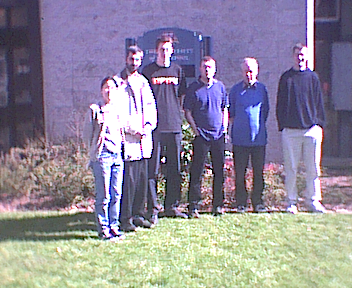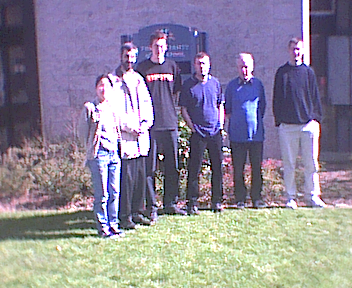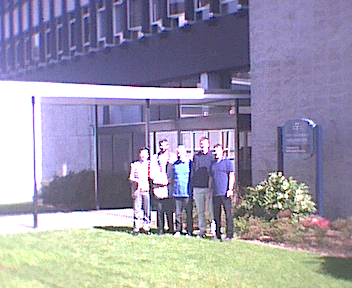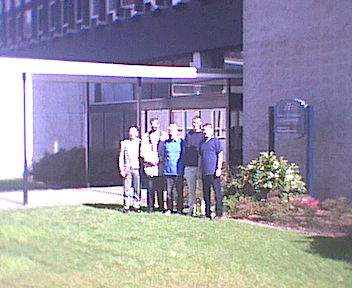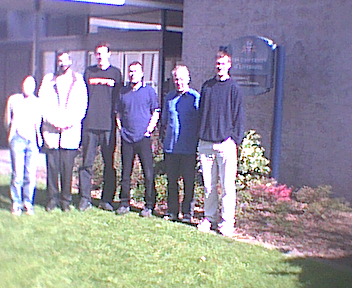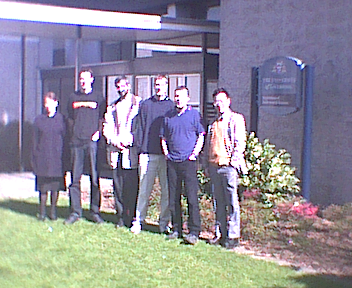## The University of Liverpool (maps) Division of Applied Mathematics Department of Mathematical Sciences

Tel: +44 (0) 151 794 4001 (Liverpool L69 7ZL, England) Fax: +44 (0)151 794 4061

```

```
Recent PhD Thesis from CMIT: